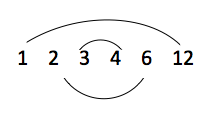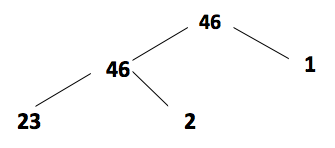What is a factor? Factors are whole numbers that divide exactly into other whole numbers.

For example:

The factors of 12 are: 1, 2, 3, 4, 6 and 12.

1 x 12 = 12

2 x 6 = 12

3 x 4 = 12

We can then say that 12 is a multiple of 1, 2, 3, 4, 6 and 12.

Multiples can be thought of as extended times tables, for example: the multiples of 8 are all the numbers in the 8 times table: 8, 16, 32, 64, 128 etc.

Factors come in pairs that multiply together to form a certain number, this is easily demonstrated in a rainbow diagram like below:This shows the pairs of factors which multiply to equal 12.

A helpful way for children to visualise the factors of a number is by drawing factor trees. These diagrams are also useful when children are asked to look for factors that different numbers have in common. Here is a factor tree for the number 46:## When do children learn about factors?

Children are actually learning about factors from Year 1 onwards when they start learning to count. Learning times tables is steadily introduced throughout Years 2 and 3, and in Year 4 children are expected to have learnt all the times tables up to 12.

In Year 4 the term ‘factor’ will be used, and children may have practice questions to complete like the example below:

Out of the following numbers, find two pairs of factors which multiply to equal 42

3 x 14     9 x 5     2 x 24     6 x 7

The answers are: 3 x 14 and 6 x 7.

## How does a knowledge of factors help children with other mathematical concepts?

A good knowledge of factors is helpful for children in Year 5 onwards, as this is when they progress onto learning about prime numbers and square numbers. Children also need to understand factors before they can go on to learn about the highest common factor.

In Year 6 children should be confident in their knowledge of times tables and factors, as they will be expected to answer more complex questions and puzzles.

An example question might be:

I am a number less than 40. The sum of my digits is 8 and one of my factors is 7. What number am I?

To find the answer to this puzzle, children can use their knowledge of the 7 times table. They may write down all the multiples of 7 which are less than 40:

7, 14, 21, 28 and 35

Then they can see which of these numbers has digits that equal 8. As 3 + 5 = 8, the answer is 35.

## How does Learning Street help children with factors?

Learning Street will introduce factors to the children slowly. This will be combined with regular practice in order that it is understood before then beginning to develop their knowledge and extend it further, as well as constant revision so that it isn’t forgotten.

## Our Courses

Click through to review the courses we have available

##### Get Started

Learning Street structured courses make home study easier and more successful. There's no need to wonder which books to buy or what to do next. Everything is planned for you.

• Proven to deliver excellent results
• Used by families, private tutors and schools
• Fully planned and structured